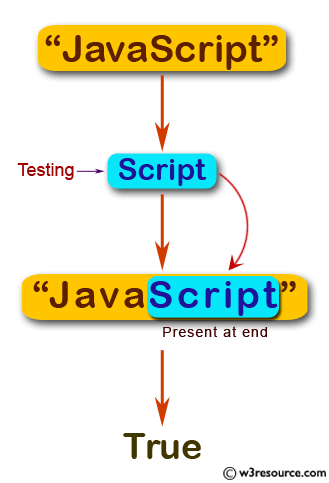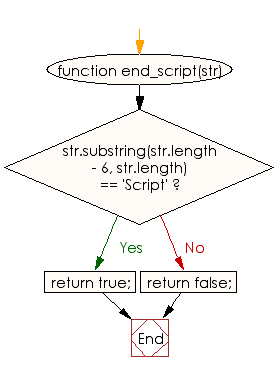# JavaScript: Test whether a string end with "Script"

## JavaScript Basic: Exercise-65 with Solution

Write a JavaScript program to test whether a string end with "Script". The string length must be greater or equal to 6.

Pictorial Presentation:Sample Solution:

HTML Code:

``````<!DOCTYPE html>
<html>
<meta charset="utf-8">
<meta name="viewport" content="width=device-width">
<title>JavaScript program to test whether a string end with “Script”.  The string length must be greater or equal to 6.</title>
<body>

</body>
</html>
```
```

JavaScript Code:

``````function end_script(str) {
if (str.substring(str.length - 6, str.length) == 'Script')
{
return true;
}
else
{
return false;
}
}
console.log(end_script("JavaScript"));
console.log(end_script("Java Script"));
console.log(end_script("Java Scripts"));
```
```

Sample Output:

```true
true
false
```

Flowchart:ES6 Version:

``````function end_script(str) {
if (str.substring(str.length - 6, str.length) == 'Script')
{
return true;
}
else
{
return false;
}
}
console.log(end_script("JavaScript"));
console.log(end_script("Java Script"));
console.log(end_script("Java Scripts"));
``````

Live Demo:

See the Pen JavaScript - Test if a string end with Script - basic-ex-65 by w3resource (@w3resource) on CodePen.

What is the difficulty level of this exercise?

Test your Programming skills with w3resource's quiz.

﻿

## JavaScript: Tips of the Day

Checks if a string is an anagram of another string (case-insensitive, ignores spaces, punctuation and special characters)

Example:

```const isAnagram = (str1, str2) => {
const normalize = str =>
str
.toLowerCase()
.replace(/[^a-z0-9]/gi, '')
.split('')
.sort()
.join('');
return normalize(str1) === normalize(str2);
};
console.log(isAnagram('iceman', 'cinema')); // true
```

Output:

```true
```